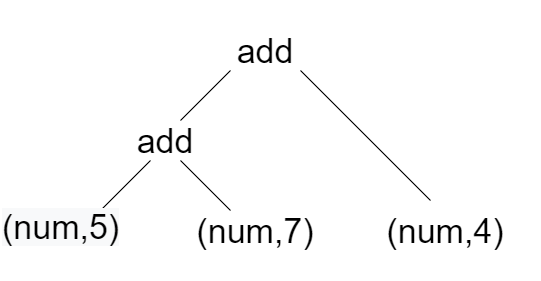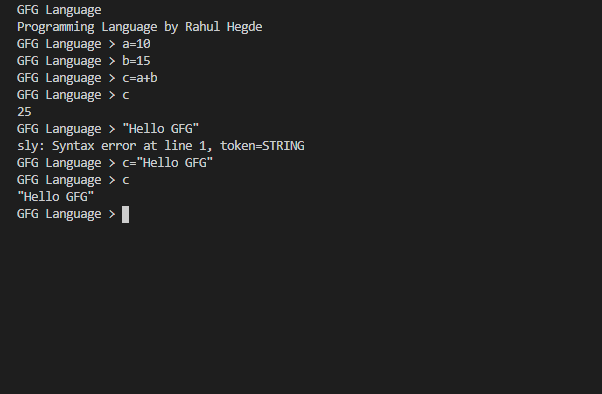# How to Create a Programming Language using Python?

In this article, we are going to learn how to create your own programming language using SLY(Sly Lex Yacc) and Python. Before we dig deeper into this topic, it is to be noted that this is not a beginner’s tutorial and you need to have some knowledge of the prerequisites given below.

#### Prerequisites

• Rough knowledge about compiler design.
• Basic understanding of lexical analysis, parsing and other compiler design aspects.
• Understanding of regular expressions.
• Familiarity with Python programming language.

## Getting Started

Install SLY for Python. SLY is a lexing and parsing tool which makes our process much easier.

```pip install sly
```

## Building a Lexer

The first phase of a compiler is to convert all the character streams(the high level program that is written) to token streams. This is done by a process called lexical analysis. However, this process is simplified by using SLY

First let’s import all the necessary modules.

## Python3

 `from` `sly ``import` `Lexer`

Now let’s build a class BasicLexer which extends the Lexer class from SLY.  Let’s make a compiler that makes simple arithmetic operations. Thus we will need some basic tokens such as NAME, NUMBER, STRING. In any programming language, there will be space between two characters. Thus we create an ignore literal. Then we also create the basic literals like ‘=’, ‘+’ etc., NAME tokens are basically names of variables, which can be defined by the regular expression [a-zA-Z_][a-zA-Z0-9_]*. STRING tokens are string values and are bounded by quotation marks(” “). This can be defined by the regular expression \”.*?\”.

Whenever we find digit/s, we should allocate it to the token NUMBER and the number must be stored as an integer. We are doing a basic programmable script, so let’s just make it with integers, however, feel free to extend the same for decimals, long etc., We can also make comments. Whenever we find “//”, we ignore whatever that comes next in that line. We do the same thing with new line character. Thus, we have build a basic lexer that converts the character stream to token stream.

## Python3

 `class` `BasicLexer(Lexer): ` `    ``tokens ``=` `{ NAME, NUMBER, STRING } ` `    ``ignore ``=` `'\t '` `    ``literals ``=` `{ ``'='``, ``'+'``, ``'-'``, ``'/'``,  ` `                ``'*'``, ``'('``, ``')'``, ``','``, ``';'``} ` ` `  ` `  `    ``# Define tokens as regular expressions ` `    ``# (stored as raw strings) ` `    ``NAME ``=` `r``'[a-zA-Z_][a-zA-Z0-9_]*'` `    ``STRING ``=` `r``'\".*?\"'` ` `  `    ``# Number token ` `    ``@_``(r``'\d+'``) ` `    ``def` `NUMBER(``self``, t): ` `       `  `        ``# convert it into a python integer ` `        ``t.value ``=` `int``(t.value)  ` `        ``return` `t ` ` `  `    ``# Comment token ` `    ``@_``(r``'//.*'``) ` `    ``def` `COMMENT(``self``, t): ` `        ``pass` ` `  `    ``# Newline token(used only for showing ` `    ``# errors in new line) ` `    ``@_``(r``'\n+'``) ` `    ``def` `newline(``self``, t): ` `        ``self``.lineno ``=` `t.value.count(``'\n'``)`

## Building a Parser

First let’s import all the necessary modules.

## Python3

 `from` `sly ``import` `Parser`

Now let’s build a class BasicParser which extends the Lexer class. The token stream from the BasicLexer is passed to a variable tokens. The precedence is defined, which is the same for most programming languages. Most of the parsing written in the program below is very simple. When there is nothing, the statement passes nothing. Essentially you can press enter on your keyboard(without typing in anything) and go to the next line. Next, your language should comprehend assignments using the “=”.  This is handled in line 18 of the program below. The same thing can be done when assigned to a string.

## Python3

 `class` `BasicParser(Parser): ` `    ``#tokens are passed from lexer to parser ` `    ``tokens ``=` `BasicLexer.tokens ` ` `  `    ``precedence ``=` `( ` `        ``(``'left'``, ``'+'``, ``'-'``), ` `        ``(``'left'``, ``'*'``, ``'/'``), ` `        ``(``'right'``, ``'UMINUS'``), ` `    ``) ` ` `  `    ``def` `__init__(``self``): ` `        ``self``.env ``=` `{ } ` ` `  `    ``@_``('') ` `    ``def` `statement(``self``, p): ` `        ``pass` ` `  `    ``@_``(``'var_assign'``) ` `    ``def` `statement(``self``, p): ` `        ``return` `p.var_assign ` ` `  `    ``@_``(``'NAME "=" expr'``) ` `    ``def` `var_assign(``self``, p): ` `        ``return` `(``'var_assign'``, p.NAME, p.expr) ` ` `  `    ``@_``(``'NAME "=" STRING'``) ` `    ``def` `var_assign(``self``, p): ` `        ``return` `(``'var_assign'``, p.NAME, p.STRING) ` ` `  `    ``@_``(``'expr'``) ` `    ``def` `statement(``self``, p): ` `        ``return` `(p.expr) ` ` `  `    ``@_``(``'expr "+" expr'``) ` `    ``def` `expr(``self``, p): ` `        ``return` `(``'add'``, p.expr0, p.expr1) ` ` `  `    ``@_``(``'expr "-" expr'``) ` `    ``def` `expr(``self``, p): ` `        ``return` `(``'sub'``, p.expr0, p.expr1) ` ` `  `    ``@_``(``'expr "*" expr'``) ` `    ``def` `expr(``self``, p): ` `        ``return` `(``'mul'``, p.expr0, p.expr1) ` ` `  `    ``@_``(``'expr "/" expr'``) ` `    ``def` `expr(``self``, p): ` `        ``return` `(``'div'``, p.expr0, p.expr1) ` ` `  `    ``@_``(``'"-" expr %prec UMINUS'``) ` `    ``def` `expr(``self``, p): ` `        ``return` `p.expr ` ` `  `    ``@_``(``'NAME'``) ` `    ``def` `expr(``self``, p): ` `        ``return` `(``'var'``, p.NAME) ` ` `  `    ``@_``(``'NUMBER'``) ` `    ``def` `expr(``self``, p): ` `        ``return` `(``'num'``, p.NUMBER)`

The parser should also parse in arithmetic operations, this can be done by expressions. Let’s say you want something like shown below. Here all of them are made into token stream line-by-line and parsed line-by-line. Therefore, according to the program above, a = 10 resembles line 22. Same for b =20. a + b resembles line 34, which returns a parse tree (‘add’, (‘var’, ‘a’), (‘var’, ‘b’)).

```GFG Language > a = 10
GFG Language > b = 20
GFG Language > a + b
30
```

Now we have converted the token streams to a parse tree. Next step is to interpret it.

## Execution

Interpreting is a simple procedure. The basic idea is to take the tree and walk through it to and evaluate arithmetic operations hierarchically. This process is recursively called over and over again till the entire tree is evaluated and the answer is retrieved. Let’s say, for example, 5 + 7 + 4. This character stream is first tokenized to token stream in a lexer. The token stream is then parsed to form a parse tree. The parse tree essentially returns (‘add’, (‘add’, (‘num’, 5), (‘num’, 7)), (‘num’, 4)). (see image below)The interpreter is going to add 5 and 7 first and then recursively call walkTree and add 4 to the result of addition of 5 and 7. Thus, we are going to get 16. The below code does the same process.

## Python3

 `class` `BasicExecute: ` `   `  `    ``def` `__init__(``self``, tree, env): ` `        ``self``.env ``=` `env ` `        ``result ``=` `self``.walkTree(tree) ` `        ``if` `result ``is` `not` `None` `and` `isinstance``(result, ``int``): ` `            ``print``(result) ` `        ``if` `isinstance``(result, ``str``) ``and` `result[``0``] ``=``=` `'"'``: ` `            ``print``(result) ` ` `  `    ``def` `walkTree(``self``, node): ` ` `  `        ``if` `isinstance``(node, ``int``): ` `            ``return` `node ` `        ``if` `isinstance``(node, ``str``): ` `            ``return` `node ` ` `  `        ``if` `node ``is` `None``: ` `            ``return` `None` ` `  `        ``if` `node[``0``] ``=``=` `'program'``: ` `            ``if` `node[``1``] ``=``=` `None``: ` `                ``self``.walkTree(node[``2``]) ` `            ``else``: ` `                ``self``.walkTree(node[``1``]) ` `                ``self``.walkTree(node[``2``]) ` ` `  `        ``if` `node[``0``] ``=``=` `'num'``: ` `            ``return` `node[``1``] ` ` `  `        ``if` `node[``0``] ``=``=` `'str'``: ` `            ``return` `node[``1``] ` ` `  `        ``if` `node[``0``] ``=``=` `'add'``: ` `            ``return` `self``.walkTree(node[``1``]) ``+` `self``.walkTree(node[``2``]) ` `        ``elif` `node[``0``] ``=``=` `'sub'``: ` `            ``return` `self``.walkTree(node[``1``]) ``-` `self``.walkTree(node[``2``]) ` `        ``elif` `node[``0``] ``=``=` `'mul'``: ` `            ``return` `self``.walkTree(node[``1``]) ``*` `self``.walkTree(node[``2``]) ` `        ``elif` `node[``0``] ``=``=` `'div'``: ` `            ``return` `self``.walkTree(node[``1``]) ``/` `self``.walkTree(node[``2``]) ` ` `  `        ``if` `node[``0``] ``=``=` `'var_assign'``: ` `            ``self``.env[node[``1``]] ``=` `self``.walkTree(node[``2``]) ` `            ``return` `node[``1``] ` ` `  `        ``if` `node[``0``] ``=``=` `'var'``: ` `            ``try``: ` `                ``return` `self``.env[node[``1``]] ` `            ``except` `LookupError: ` `                ``print``(``"Undefined variable '"``+``node[``1``]``+``"' found!"``) ` `                ``return` `0`

## Displaying the Output

To display the output from the interpreter, we should write some codes. The code should first call the lexer, then the parser and then the interpreter and finally retrieves the output. The output in then displayed on to the shell.

## Python3

 `if` `__name__ ``=``=` `'__main__'``: ` `    ``lexer ``=` `BasicLexer() ` `    ``parser ``=` `BasicParser() ` `    ``print``(``'GFG Language'``) ` `    ``env ``=` `{} ` `     `  `    ``while` `True``: ` `         `  `        ``try``: ` `            ``text ``=` `input``(``'GFG Language > '``) ` `         `  `        ``except` `EOFError: ` `            ``break` `         `  `        ``if` `text: ` `            ``tree ``=` `parser.parse(lexer.tokenize(text)) ` `            ``BasicExecute(tree, env)`

It is necessary to know that we haven’t handled any errors. So SLY is going to show it’s error messages whenever you do something that is not specified by the rules you have written.

Execute the program you have written using,

```python you_program_name.py
```## Footnotes

The interpreter that we built is very basic. This, of course, can be extended to do a lot more. Loops and conditionals can be added. Modular or object oriented design features can be implemented. Module integration, method definitions, parameters to methods are some of the features that can be extended on to the same.

Attention reader! Don’t stop learning now. Get hold of all the important DSA concepts with the DSA Self Paced Course at a student-friendly price and become industry ready.

My Personal Notes arrow_drop_upCheck out this Author's contributed articles.

If you like GeeksforGeeks and would like to contribute, you can also write an article using contribute.geeksforgeeks.org or mail your article to contribute@geeksforgeeks.org. See your article appearing on the GeeksforGeeks main page and help other Geeks.

Please Improve this article if you find anything incorrect by clicking on the "Improve Article" button below.

Article Tags :

Be the First to upvote.

Please write to us at contribute@geeksforgeeks.org to report any issue with the above content.